Belts and pulleys are essential power transmission components used in various applications, including material handling systems, automotive systems and gantry robots. These power transmission components convert rotary motion from one source (usually a motor) to another.

Belt and pulley transmission systems offer several advantages: they are quiet in operation, require no lubrication and are relatively cheap to produce (and maintain). But these advantages will only be felt if engineers correctly specify the motor drive for the particular application. As a rule, engineers must choose a motor that satisfies all of the torque requirements for the specific application.

This article provides helpful information about the different torque requirements in a typical motor drive for a belt and pulley transmission system. It also contains formulas for estimating the torque required for a body mass's constant velocity, acceleration and deceleration.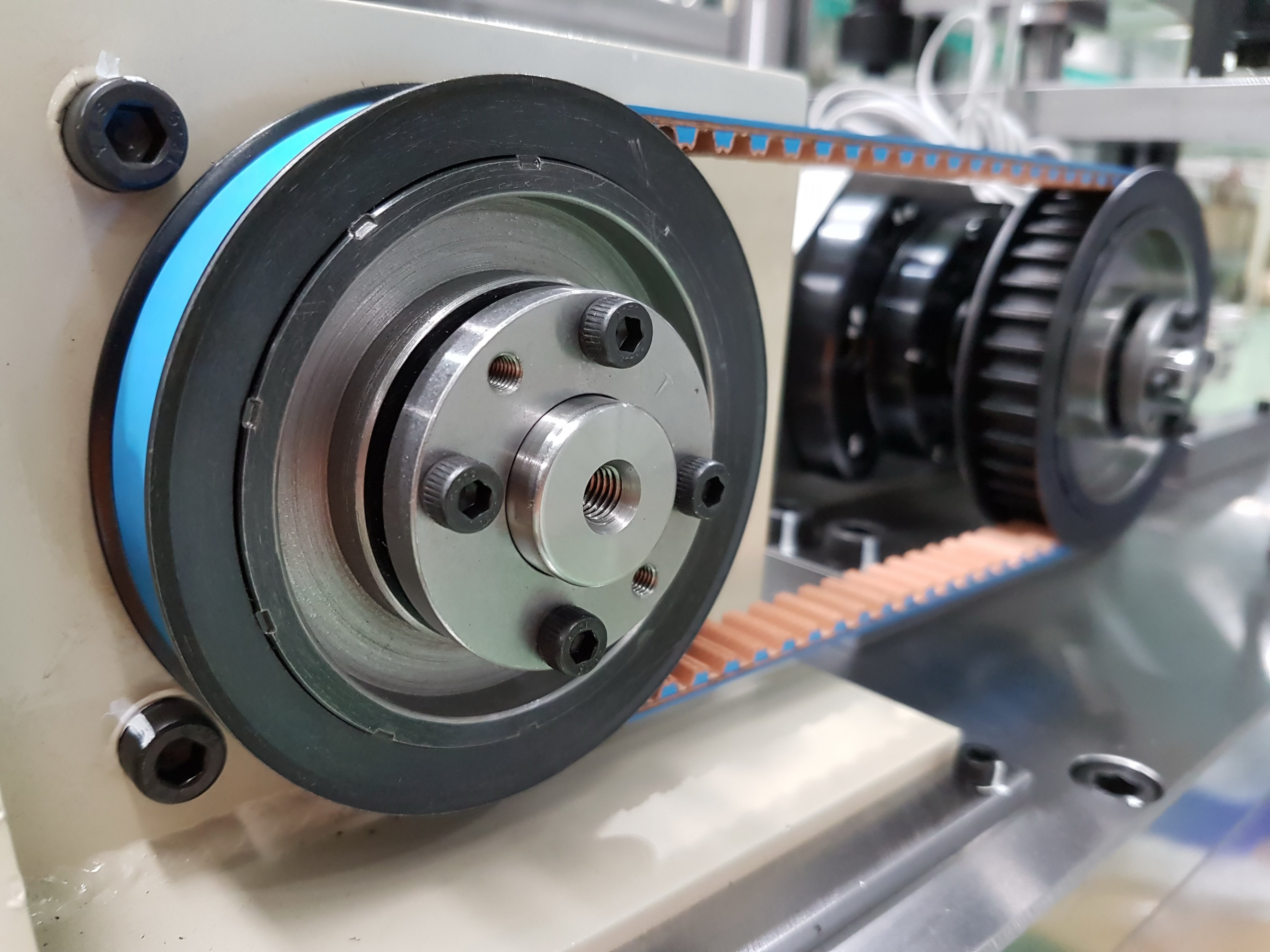Figure 1. A timing belt and timing pulley transmission system. Source: Adobe/ADS

## What is torque?

Torque describes the rotational equivalent of a linear force. It is the measure of the force that can cause an object to rotate about an axis. Torque is measured in lb-in (pounds-inch) or Nm (newton meter).

When it comes to motor drive torque for belt and pulley transmission systems, designers must understand that torque consists of two components: load torque and acceleration torque.

Load torque is the amount of torque acting on the motor due to friction and (or) gravity. For example, consider Figure 2, where a motor rotates a shaft and a pulley. The load torque can be estimated by simply multiplying the total axial force by the pulley radius.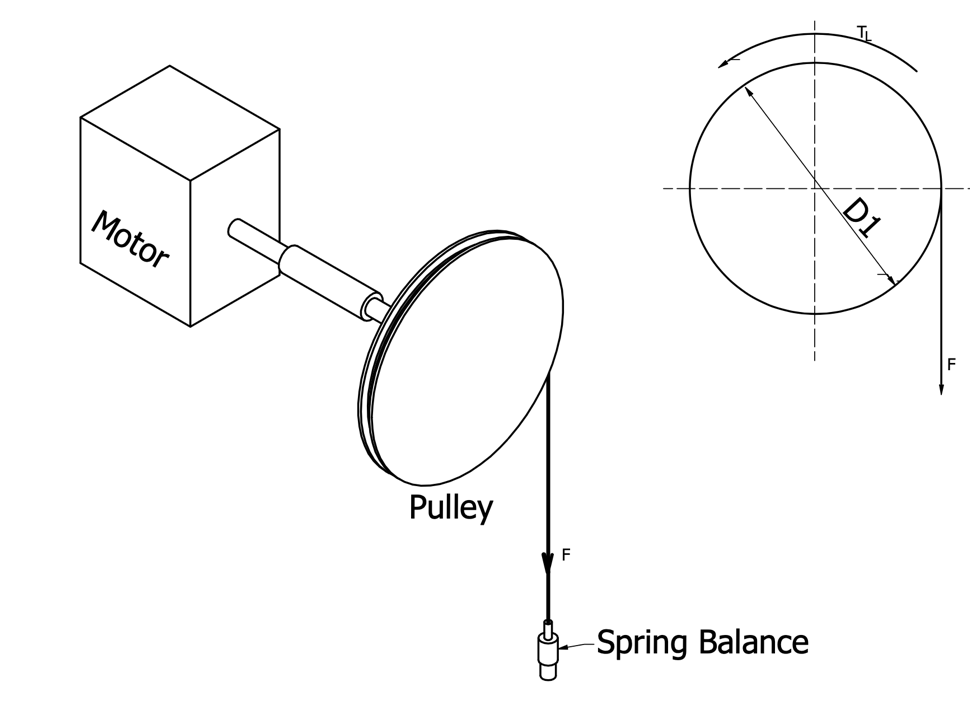Figure 2. A simple belt and pulley transmission system illustration.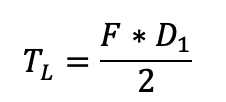Where:

F = Force when the main shaft begins to rotate

D1 = Pulley diameter

To accurately obtain the axial force value, engineers can use a spring balance to take axial force measurements. Engineers can also measure load torque by putting a torque wrench in the system.

## 2. Acceleration torque

Acceleration torque is the torque acting on the motor when it is accelerating or decelerating. It can also be described as the torque required to achieve maximum acceleration (or deceleration) of the load.

Unlike load torque, acceleration torque is extremely challenging to measure using a torque wrench. Countless engineers have horror stories about torque wrenches flying off and causing serious injury when measuring acceleration torque.

A safer way of estimating the acceleration torque is by multiplying the system's total inertia by the motor's angular acceleration.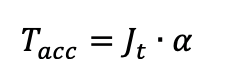Where:

Tacc = acceleration torque (Nm)

Jt = Total inertia of the system (kg/m2)

The total inertia of the system is the sum of inertia values of all the individual components, including the motor, coupling, drive and idler pulley, coupling and the load.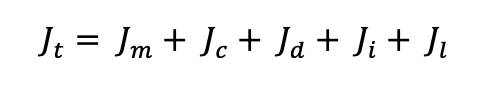Where:

Jm = Inertia of motor (kg/m2)

Jc = Inertia of the coupling (kg/m2)

Jd = Inertia of the drive pulley (kg/m2)

Ji = Inertia of idler pulley (kg/m2)

Jl = Inertia of load (kg/m2)

Manufacturers usually specify the inertia values of the motor, coupling, drive and idler pulleys. But engineers would have to calculate the inertia value of the load (which includes the external load and belt) using: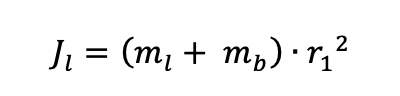torque_eq4

Where:

ml = mass of external load (kg)

mb = mass of belt (kg)

r1 = radius of drive pulley (m)

The angular acceleration (a) can simply be estimated using: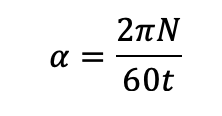torque_eq5

Where:

N = rotational speed of the motor (rpm)

t = time for acceleration (s)

## Total torque requirement

For accelerating a body, the total torque requirement that the motor must satisfy is the sum of the load torque and acceleration torque.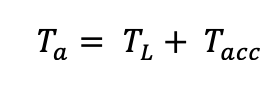torque_eq6

Where:

Ta = Total torque requirement for accelerating a body

For decelerating a body, the total torque requirement can be estimated using: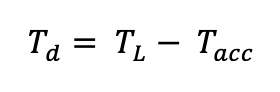torque_eq7

Where:

Td = Total torque requirement for decelerating a body.

## Conclusion

There still exist many other factors that engineers must consider when specifying motors for belt and pulley systems. For example, engineers still have to estimate power output requirements, speed ratio and type of drive.

To find suppliers to help further, or to learn more about motors, belt drives and other motion control components, visit the product areas on GlobalSpec.